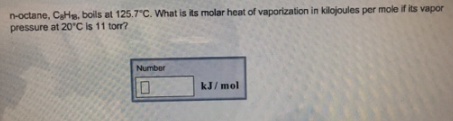# Problem: n-octane, C8H18 boils at 125.7 °C. What is its molar heat of vaporization in kilojoules per mole if its vapor pressure at 20°C is 11 torr?

###### Problem Details

n-octane, C8H18 boils at 125.7 °C. What is its molar heat of vaporization in kilojoules per mole if its vapor pressure at 20°C is 11 torr?Frequently Asked Questions

What scientific concept do you need to know in order to solve this problem?

Our tutors have indicated that to solve this problem you will need to apply the Clausius-Clapeyron Equation concept. You can view video lessons to learn Clausius-Clapeyron Equation. Or if you need more Clausius-Clapeyron Equation practice, you can also practice Clausius-Clapeyron Equation practice problems.